# Civil Engineering - UPSC Civil Service Exam Questions

41.

During the measurement of a line by chain or tape in slopes, if the length of the line is 'l' and the height difference between the ends of the line is 'h', then the correction to the measured length is more than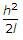by

 A. zero B.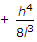C.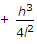D.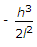Explanation:

No answer description available for this question. Let us discuss.

42.

Which one of the following represents the correct relationship between seepage pressure (ps), unit weight of water (γω) and hydraulic gradient (i), inside an earth dam ?

 A. ps = i/γω B. ps = i.γω C. ps = i2.γω D. ps = γω/i

Explanation:

No answer description available for this question. Let us discuss.

43.

Consider the following factors :
2. Large variations in stress
3. Large stress concentration.
Those associated with fatigue would include

 A. 1 and 2 B. 1 and 3 C. 2 and 3 D. 1, 2 and 3

Explanation:

No answer description available for this question. Let us discuss.

44.

As per I.S. 1904-1986 the permissible angular distortion with respect to steel sructures in isolated foundations resting on plastic clay is

 A.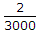B.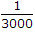C.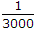D.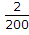Explanation:

No answer description available for this question. Let us discuss.

45.

Which one of the following statements about photogrammetric surveying is correct ? The relief displacement

 A. decreases with increase in flying height B. in negative for a point above datum C. decreases as the distance of the object from the principal point increases D. of the point is not affected by the tilt of the photograph.